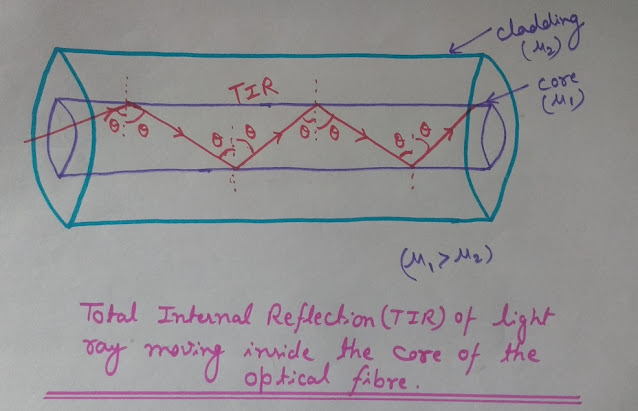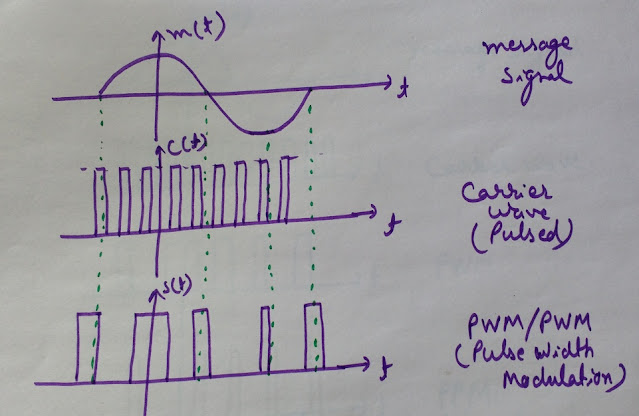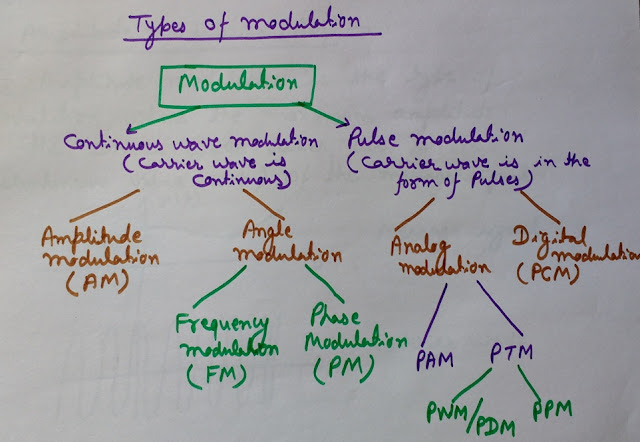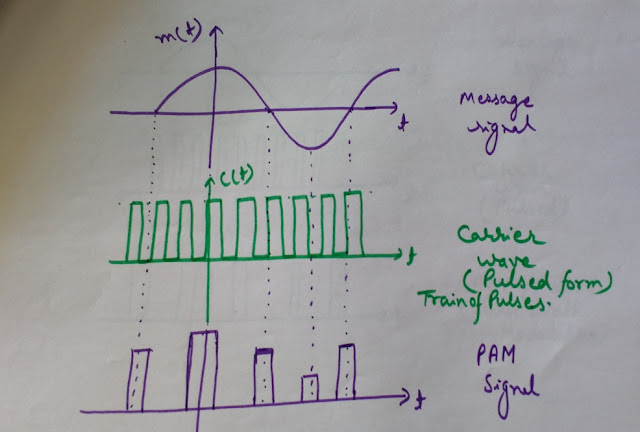## 22 Oct 2017

### Optical Fibers in Communication | All you need to know about Optical Fibers (Includes Video)

Here we will discuss all the Basics of optical fibers that include its Basic Introduction, Structure and Working Principle, Communication Process of optical fibers, Types of optical fibers, Benefits, Losses and Dispersion in optical fibers. We will discuss here each topic one by one-

# 1.What is an Optical Fiber?

An optical fiber is a flexible and transparent fiber which is made by drawing glass (silica) or plastic.
Optical fiber has a diameter slightly thicker than that of a human hair.

## 2.Structure and Working of the Optical Fiber

Optical fibers are made of glass or plastic.
Most optical fibers used in communication have diameter of 0.25 mm to 0.5 mm including outer coating.

#OPTICAL FIBER SOURCES (DESIRABLE PROPERTIES)

Optical Fiber communication takes place between 0.8 micrometer to 1.7 micrometer of wavelength of electromagnetic spectrum.
Optical fibers have a transparent core which is surrounded by a transparent cladding and the cladding has a protective covering over it.
The image given below explains the concept of Total Internal Reflection (TIR) which is the phenomenon responsible for propagation of light inside the optical fiber.Total Internal Reflection (TIR) PhenomenonTotal Internal Reflection (TIR) Inside Optical Fiber

Based on the refractive index profile, there are two categories of optical fibers-
#Step Index Optical Fibers

In step index fibers, the refractive index profile makes a step change at the core-cladding interface.
In step index fiber if core has refractive index n1 and cladding has refractive index n2, then this condition holds-
n1>n2
And this is necessary condition for Total Internal Reflection (TIR) in the optical fiber.
While the graded index fibers don't have a constant refractive index in the core but the refractive index of the core decreases with increasing radial distance from the core axis.

#STEP INDEX OPTICAL FIBER (MULTIMODE AND SINGLE MODE STEP INDEX FIBERS)

It has maximum value of refractive index at the core axis that decreases as we move away from the core axis and becomes constant in the cladding.
The light rays travel inside the core by the phenomena of total internal reflection. Since the core has higher refractive index (n1) than that of cladding (n2), i.e. n1>n2.
So when the light rays fall on the core-cladding interface (moves from denser to rarer medium), it returns back into the core.
But for the Total Internal Reflection (TIR) to take place, it is necessary for the light rays to have incidence angle greater than the critical angle while moving from denser to rarer medium (core to cladding).

## 3.Optical Fiber Communication Process

Message that we want to transmit maybe non-electrical in nature (audio signal), so first of all it needs to be converted into electrical form using transducers.
Now the message converted into electrical form modulates an optical source. Ex. LASER or LED.

#Step Index Vs Graded Index Fibers

After this the light rays containing message travel through the optical fiber by the phenomena of total internal reflection. Due to total internal reflection the energy loss is negligible inside the fiber while travelling.
Now at the receiving end, photodetectors like photodiodes or phototransistors etc., are used to convert the light signal back into electrical signal. Then the original message signal is retrieved from this electrical signal.

## 4.Types of Optical Fibers (Based on Modes of Propagation)

There are two types of optical fibers based on modes of propagation -
#Single Mode fibers (SMF)
#MultiMode fibers (MMF)
As clear by the name itself, the single mode fibers support only one propagation path, since they have very small diameter. While multimode fibers can support many propagation paths or transverse modes as they have larger diameter.
Single mode fibers are used for long distance communication while multimode fibers for short distance communication.
Single mode fiber provide greatest transmission bandwidth and lowest losses in communication.

## 5.Benefits of Optical Fibers

#Energy loss is negligible inside the optical fibers while propagation due to total internal reflection.
#Optical fibers provide very large potential bandwidth (since optical communication takes place at very high frequency (10^13-10^16).
#Optical fibres have small size, are lightweight and very flexible.

#OPTICAL FIBER: STRUCTURE AND WORKING PRINCIPLE

#Optical fibers provide electrical isolation and are shock resistant. Since inside the fiber light propagates; not any electric current.
#Optical fibers provide high degree of signal security since these fibers do not radiate significantly unlike copper cables.
#Optical fibers are easy to maintain and the communication system is reliable.

## 6.Losses in Optical Fibers

Although the optical fibers have negligible losses in propagation but some losses are still present. These losses are the following-
#Material absorption
#Linear and nonlinear scattering
#Fibre bend losses

## 7.Dispersion in optical fibers

When light rays travel through the fiber, the phenomena of dispersion (broadening of transmitted light pulses), takes place. Because of this dispersion, each pulse broadens and overlaps with its neighboring pulses. Due to this, pulses become indistinguishable at the receiving end. This effect is known as Inter Symbol Interference (ISI).

The dispersion is of two types-
#Intermodal Dispersion
#Intramodal Dispersion
Here is the comparison of intermodal dispersion in different types of optical fibers- Multimode step index fiber> Multimode graded index fiber> Single mode step index fiber

FREQUENCY SPECTRUM OF AMPLITUDE MODULATION (WAVEFORMS AND EQUATIONS DERIVATION)

AMPLITUDE MODULATION (TIME DOMAIN EQUATIONS AND WAVEFORMS)

STEP INDEX OPTICAL FIBER (MULTIMODE AND SINGLE MODE STEP INDEX FIBERS)

PULSE MODULATION TECHNIQUES (PAM, PWM, PPM, PCM)

OPTICAL FIBER: STRUCTURE AND WORKING PRINCIPLE

PULSE AMPLITUDE MODULATION (PAM)

COMPARISON OF PAM, PWM, PPM MODULATION TECHNIQUES

PULSE WIDTH MODULATION (PWM)

CONTINUOUS TIME AND DISCRETE TIME SIGNALS (C.T. AND D.T. SIGNALS)

NEED AND BENEFITS OF MODULATION

PULSE POSITION MODULATION (PPM)

OPTICAL FIBERS IN COMMUNICATION: COVERS ALL IMPORTANT POINTS

OPTICAL FIBER SOURCES (DESIRABLE PROPERTIES)

SAMPLING THEOREM AND RECONSTRUCTION (SAMPLING AND QUANTIZATION)

SUPERPOSITION THEOREM (BASICS, SOLVED PROBLEMS, APPLICATIONS AND LIMITATIONS)

Digital Modulation Techniques (ASK, FSK, PSK, BPSK)/ Amplitude, Frequency and Phase Shift Keying

Conventional AM Vs DSB-SC Vs SSB-SC Vs VSB (Comparison of AM Systems)

What are Microwaves and their Applications (Uses) in various fields

Basic Structure of Bipolar Junction Transistor (BJT) - BJT Transistor - Working and Properties

Polar Plots of Transfer Functions in Control Systems (How to Draw Nyquist Plot Examples)

Generation of Binary Phase Shift Keying (BPSK Generation) - Block Diagram of Binary Phase Shift Keying (BPSK)

Low Level and High Level Modulation Block Diagram (AM Transmitter Block Diagram)

Block Diagram of CRO (Cathode Ray Oscilloscope), Components of CRO and CRT with Structure and Working

Slope Overload Distortion and Granular (Idle Noise), Quantization Noise in Delta Modulation

Frequency Translation/Frequency Mixing/Frequency Conversion/Heterodyning (Basic Concepts and Need)

Quadrature Phase Shift Keying Modulation (QPSK) Basics, Waveform and Benefits

Pulse Code Modulation (PCM) Vs Differential Pulse Code Modulation (DPCM)

# What is a Signal?

## Definition of Signal

A signal may be defined as a function of one or more independent variables like time, distance, position or temperature etc., that has some information about the phenomena that produced the signal.

COMPARISON OF PAM, PWM, PPM MODULATION TECHNIQUES

So in other words we can say, a signal is a function of independent variables having some information.
The signals maybe motion signals, sound signals, image signals or video signals etc.

# Continuous Time and Discrete Time Signals Video [HD]

Signals can be classified into two types based on their characteristics in time domain-
Continuous Time Signals (C.T.)
Discrete Time Signals (D.T.)

# Continuous Time Signals (C.T.)

A signal that is defined continuously with independent variable (time) is called a continuous time signal.

### What is Modulation and why Modulation is Required | Need and Benefits of Modulation

Before understanding the need and benefits of modulation, it is important to know, what is modulation?

# What is Modulation

Modulation is a process by which some characteristic (amplitude/frequency/phase) of a high frequency carrier wave is varied, in accordance with the instantaneous value of the message signal (modulating signal/ baseband signal).

COMPARISON OF PAM, PWM, PPM MODULATION TECHNIQUES

Now let's write down the benefits of modulation. We will discuss each point in detail one by one-

# Need and Benefits of Modulation

1.To remove interference problem
2.Practical height of the antenna
3.Possibility of multiplexing
4.Long distance communication
5.Improved signal quality at the receiver

## 1.To Remove Interference Problem

Modulation helps in removing the interference problem by allocating a separate frequency band to different signal transmissions.
Because of this it becomes possible for many stations to transmit their message signals simultaneously without any interference.
Ex. The transmission range for audio signals is 20Hz-20kHz.

The height of antenna used for transmission and reception of the signal also depends on the process of modulation. The transmitting and receiving Antennas must have lengths, comparable to a quarter wavelength (λ/4) of the frequency used for transmission.

#Digital Modulation Techniques (ASK, FSK, PSK, BPSK)/ Amplitude, Frequency and Phase Shift Keying

To understand how the frequency used for transmission of the signal affects the height of the antenna.
As mentioned earlier, height of the antenna should be comparable to λ/4 of the frequency used for transmission.
height of the antenna= λ/4
Since we know that-
c=λ.f
here c is speed of light in vacuum= 3×10^8 m/s
f is frequency
Therefore λ= c/f
So height of the antenna would be-
(c/f).(1/4)
here c and 1/4 are constants
Therefore antenna height is inversely proportional to frequency used for transmission.
It means as the frequency of modulation increases, height of the antenna used for transmission decreases.
We Will understand it will the help of an example-
Here we will see, how the height of the antenna reduced when we increased the frequency used for transmission from 4kHz to 5MHz after modulation.
Height= λ/4
=(c/f).(1/4)
= (3×10^8 m/s)/(4×10^3Hz)× (1/4)
=0.187×10^5 meters
= 18.7 kilometers !!!
This height of antenna is not practical.
After modulation-
Frequency used is 5MHz
H=(c/f).(1/4)
=(3×10^8 m/s)/(5×10^6Hz)× (1/4)
=0.15×10^2 meters
15meters
It is practical height of the antenna.

Multiplexing can be Defined as a process by which two or more signals can be transmitted simultaneously over the same channel for communication.
Modulation can be used to allocate separate frequency bands by using career waves of different frequencies. This type of multiplexing is known as Frequency division multiplexing (FDM).

## 4.Long Distance Communication

Modulation is used for long distance communication. In modulation we use high frequency carrier wave for transmission of the message signal.

High frequency signals attenuate less as compared to low frequency signals. Therefore moderation is beneficial for long distance communication.

### PULSE POSITION MODULATION (PPM) - Basics, Definition and Waveform of PPM

Pulse modulation can be categorized broadly into two types-
#Analog modulation and
#Digital modulation
The analog pulse modulation can again be of two types-

# Pulse Position Modulation Video [HD]

#Pulse Amplitude Modulation (PAM)
#Pulse Time Modulation (PTM)
The Pulse Time Modulation (PTM) can further be classified into two types of modulation-
#Pulse Width Modulation (PWM)/Pulse Duration Modulation (PDM)
#Pulse Position Modulation (PPM)
Pulse Code Modulation (PCM) is a digital pulse modulation technique.

# What is Pulse Modulation

Pulse modulation is a type of modulation where some parameter of the pulsed carrier wave is varied as per the instantaneous value of the modulating signal (message signal).

## Types of Pulse Modulation Techniques

Pulse modulation can be categorized into 3 types-

2.Pulse Width Modulation (PWM)

Out of these three types of pulse modulation techniques, PWM and PPM come under Pulse Time Modulation (PTM).

# Pulse Width Modulation Video [HD]

## Pulse Time Modulation (PTM)

In pulse time modulation, the timing of pulses of the pulsed carrier is varied. So the variations occur on the time axis. Since it is of two types, Pulse Width Modulation and Pulse Position Modulation, where width and position of the pulses is varied respectively. These changes in width or position take place on the time axis. Therefore PWM and PPM are types of pulse time modulation.

COMPARISON OF PAM, PWM, PPM MODULATION TECHNIQUES

Now we will understand the Pulse Width Modulation (PWM), in detail-

### Definition:-

In Pulse width modulation, width (duration) of the pulses of the carrier wave is varied according to the modulating signal (message signal).
Let's analyze the waveform of pulse width modulation.
Look at the image given below to see the waveform of pulse width modulated signal. (Click the image to enlarge)-Pulse Width Modulation Waveform (PWM)

Here you can see waveforms of modulating signal, pulsed carrier wave and pulse width modulated wave.

As per the definition of pulse width modulation, we know that, in Pulse width modulation, the width of the pulses of carrier wave is varied according to the message signal. So you can see here in the image that as the amplitude of the message signal changes, the width of pulses of the pulsed carrier wave changes accordingly.
You can easily observe here, the width of the pulse is maximum when amplitude of the message signal is at maximum and in the same way, width is minimum when amplitude of the modulating signal is minimum.

1.Since it is pulse width modulation (pulse duration modulation), therefore only the width (duration) of the pulses of the carrier wave changes. No change takes place in amplitude or position of the pulses.

#Watch the VIDEOS PLAYLIST here (Pulse modulation Techniques)

2.As the width of pulses changes according to the modulating signal; information is present only in the width of the pulses. In Pulse width modulation, amplitude or position of the pulses contain no information.

## Properties of Pulse Width Modulation (PWM)

#### 1.Power in PWM

In PWM, instantaneous power of the transmitter varies due to variations in width of the pulses.

#### 2.Noise in PWM

Pulse Width Modulation is less affected by noise due to its constant amplitude. It is also easier to remove noise from the signal as compared to Pulse amplitude modulation.

## 14 Oct 2017

### PULSE AMPLITUDE MODULATION (PAM) || Definition, Basics and Waveform of PAM Explained

In this post we will discuss Pulse Amplitude Modulation (PAM). Pulse Amplitude Modulation is a pulse modulation technique. Other techniques of analog pulse modulation are- Pulse Width Modulation (PWM) and Pulse Position Modulation (PPM). But before discussing pulse amplitude modulation, it is important to know, what is pulse modulation and how it is different from continuous wave modulation.

#NEED AND BENEFITS OF MODULATION

# Difference between Pulse Modulation and Continuous Wave Modulation

In pulse modulation, some parameter (amplitude, width or position) of the pulsed carrier wave is varied as per the instantaneous value of the modulating signal (message signal). Types of analog pulse modulation techniques are- Pulse Amplitude Modulation (PAM), Pulse Width Modulation (PWM) and Pulse Position Modulation (PPM).
While continuous wave modulation is a technique of modulation where some parameter (amplitude, frequency or phase) of a sinusoidal carrier is varied in accordance with the instantaneous value of the  modulating signal.

COMPARISON OF PAM, PWM, PPM MODULATION TECHNIQUES

Types of continuous wave modulation are- Amplitude modulation (AM), Frequency Modulation (FM) and Phase Modulation (PM).
See the image given below to understand different types of modulation techniques. Click the image to enlarge it-

##Classification of Modulation Techniques

The definitions of PAM, PWM and PPM are given below for quick reference-

## Pulse Amplitude Modulation (PAM)

In pulse amplitude modulation, the amplitude of pulsed carrier wave is varied according to the modulating signal.

## Pulse Time Modulation (PTM)

In pulse time modulation, the timing of pulses of the pulsed carrier is varied. Therefore the variations in the pulse are on the time axis.

Pulse Time Modulation (PTM) is of two types-
Pulse Position Modulation (PPM)

## Pulse Width Modulation (PWM)

In PWM, the width of pulsed carrier wave is varied as per the instantaneous value of the modulating signal.

## Pulse Position Modulation (PPM)

In PPM, the position of pulsed carrier is varied as per the modulating signal (message signal).

Now we understand the basic difference between PAM, PWM and PPM. Now it's time to discuss pulse Amplitude Modulation in some detail-

# Pulse Amplitude Modulation (PAM)

## Definition:-

The modulation technique in which the instantaneous amplitude of the pulsed carrier is varied according to the modulating signal (message signal) is called as pulse amplitude modulation (PAM).

## Waveform of Pulse Amplitude Modulation (PAM)

Look at the image given below carefully to understand the formation of pulse amplitude modulated wave. (Click the image to enlarge)-Pulse Amplitude Modulation (PAM) Waveform

Here in this image, you can see, three waveforms.

#SAMPLING THEOREM AND RECONSTRUCTION (SAMPLING AND QUANTIZATION)
#Watch the VIDEOS PLAYLIST here (Pulse modulation Techniques)

The first waveform is of modulating signal (message signal), the second one is of the pulsed career wave and the third waveform is of the pulse amplitude modulated wave (PAM).
The message signal can have multiple frequencies and variable amplitude. Here we have used a sinusoidal message signal. The carrier wave that we have used here, is a train of pulses having high frequency. Since we know that in Pulse modulation, the carrier wave is in the form of pulses.
Now we will understand how can we make pulse amplitude modulated wave (PAM).

#AMPLITUDE MODULATION (TIME DOMAIN EQUATIONS AND WAVEFORMS)
#FREQUENCY SPECTRUM OF AMPLITUDE MODULATION (WAVEFORMS AND EQUATIONS DERIVATION)

As we have discussed that in Pulse amplitude modulation, the instantaneous amplitude of the pulsed carrier is varied according to the modulating signal (message signal). Now look at the image shown above very carefully, observe that, as the amplitude of the modulating signal (message signal or baseband signal) increases, the amplitude of the pulsed carrier wave increases and becomes maximum when the amplitude of the modulating signal reaches at its maximum. Now the amplitude of the carrier wave starts decreasing with decrease in amplitude of the modulating signal. So it is clear from the image that the amplitude of the pulsed carrier wave varies according to the instantaneous value of the message signal.

## Important Observations about Pulse Amplitude Modulation (PAM)

#As it is Pulse Amplitude Modulation (PAM), therefore only the amplitude of the pulsed carrier changes.
#There is no change in the width or position of the pulsed carrier wave. So the width and position of the carrier wave pulse is constant here.